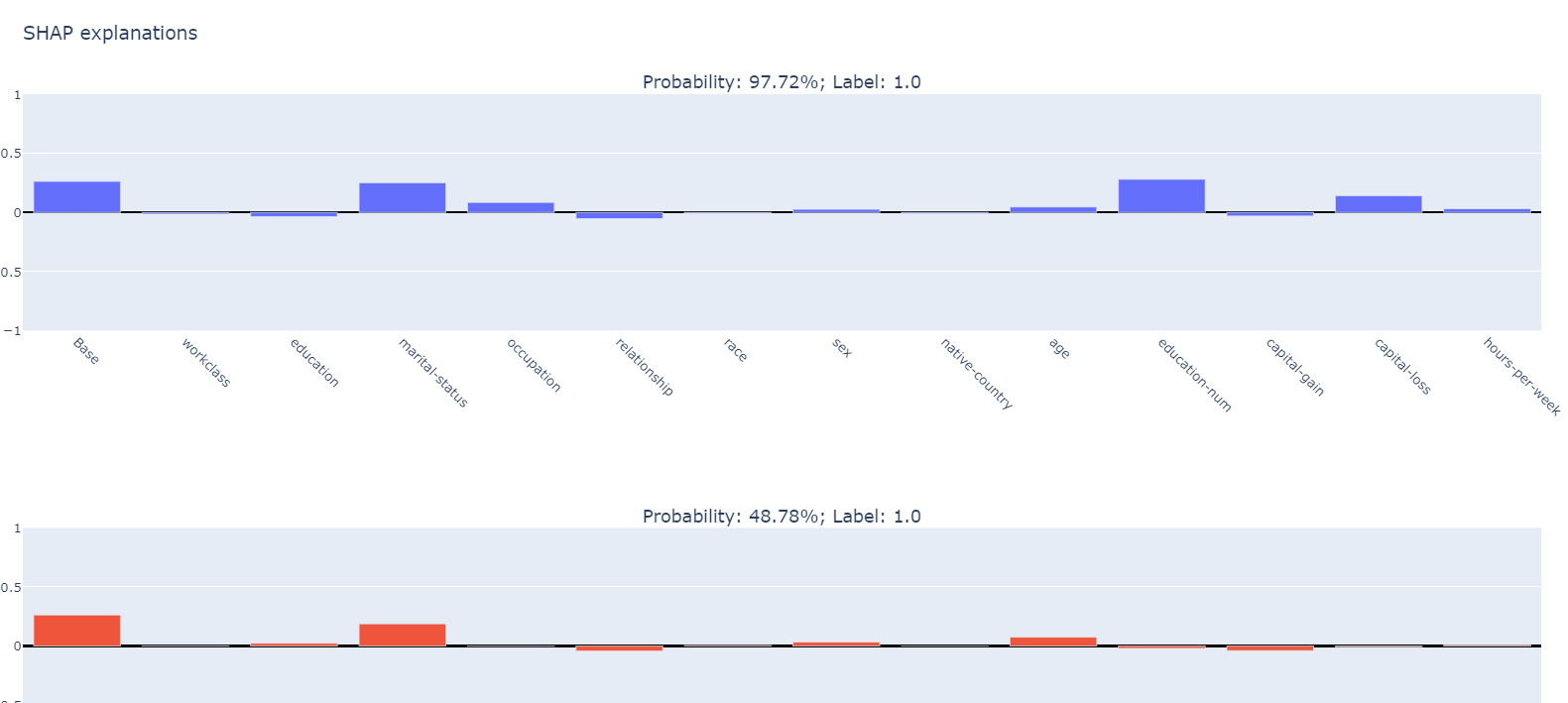Version: 0.9.5

## Interpretability - Tabular SHAP explainer​

In this example, we use Kernel SHAP to explain a tabular classification model built from the Adults Census dataset.

First we import the packages and define some UDFs we will need later.

import pysparkfrom synapse.ml.explainers import *from pyspark.ml import Pipelinefrom pyspark.ml.classification import LogisticRegressionfrom pyspark.ml.feature import StringIndexer, OneHotEncoder, VectorAssemblerfrom pyspark.sql.types import *from pyspark.sql.functions import *import pandas as pdvec_access = udf(lambda v, i: float(v[i]), FloatType())vec2array = udf(lambda vec: vec.toArray().tolist(), ArrayType(FloatType()))

Now let's read the data and train a simple binary classification model.

df = spark.read.parquet("wasbs://publicwasb@mmlspark.blob.core.windows.net/AdultCensusIncome.parquet")labelIndexer = StringIndexer(inputCol="income", outputCol="label", stringOrderType="alphabetAsc").fit(df)print("Label index assigment: " + str(set(zip(labelIndexer.labels, [0, 1]))))training = labelIndexer.transform(df).cache()display(training)categorical_features = [    "workclass",    "education",    "marital-status",    "occupation",    "relationship",    "race",    "sex",    "native-country",]categorical_features_idx = [col + "_idx" for col in categorical_features]categorical_features_enc = [col + "_enc" for col in categorical_features]numeric_features = ["age", "education-num", "capital-gain", "capital-loss", "hours-per-week"]strIndexer = StringIndexer(inputCols=categorical_features, outputCols=categorical_features_idx)onehotEnc = OneHotEncoder(inputCols=categorical_features_idx, outputCols=categorical_features_enc)vectAssem = VectorAssembler(inputCols=categorical_features_enc + numeric_features, outputCol="features")lr = LogisticRegression(featuresCol="features", labelCol="label", weightCol="fnlwgt")pipeline = Pipeline(stages=[strIndexer, onehotEnc, vectAssem, lr])model = pipeline.fit(training)

After the model is trained, we randomly select some observations to be explained.

explain_instances = model.transform(training).orderBy(rand()).limit(5).repartition(200).cache()display(explain_instances)

We create a TabularSHAP explainer, set the input columns to all the features the model takes, specify the model and the target output column we are trying to explain. In this case, we are trying to explain the "probability" output which is a vector of length 2, and we are only looking at class 1 probability. Specify targetClasses to [0, 1] if you want to explain class 0 and 1 probability at the same time. Finally we sample 100 rows from the training data for background data, which is used for integrating out features in Kernel SHAP.

shap = TabularSHAP(    inputCols=categorical_features + numeric_features,    outputCol="shapValues",    numSamples=5000,    model=model,    targetCol="probability",    targetClasses=,    backgroundData=broadcast(training.orderBy(rand()).limit(100).cache()),)shap_df = shap.transform(explain_instances)

Once we have the resulting dataframe, we extract the class 1 probability of the model output, the SHAP values for the target class, the original features and the true label. Then we convert it to a pandas dataframe for visisualization. For each observation, the first element in the SHAP values vector is the base value (the mean output of the background dataset), and each of the following element is the SHAP values for each feature.

shaps = (    shap_df.withColumn("probability", vec_access(col("probability"), lit(1)))    .withColumn("shapValues", vec2array(col("shapValues").getItem(0)))    .select(["shapValues", "probability", "label"] + categorical_features + numeric_features))shaps_local = shaps.toPandas()shaps_local.sort_values("probability", ascending=False, inplace=True, ignore_index=True)pd.set_option("display.max_colwidth", None)shaps_local

We use plotly subplot to visualize the SHAP values.

from plotly.subplots import make_subplotsimport plotly.graph_objects as goimport pandas as pdfeatures = categorical_features + numeric_featuresfeatures_with_base = ["Base"] + featuresrows = shaps_local.shapefig = make_subplots(    rows=rows,    cols=1,    subplot_titles="Probability: " + shaps_local["probability"].apply("{:.2%}".format) + "; Label: " + shaps_local["label"].astype(str),)for index, row in shaps_local.iterrows():    feature_values =  + [row[feature] for feature in features]    shap_values = row["shapValues"]    list_of_tuples = list(zip(features_with_base, feature_values, shap_values))    shap_pdf = pd.DataFrame(list_of_tuples, columns=["name", "value", "shap"])    fig.add_trace(        go.Bar(x=shap_pdf["name"], y=shap_pdf["shap"], hovertext="value: " + shap_pdf["value"].astype(str)),        row=index + 1,        col=1,    )fig.update_yaxes(range=[-1, 1], fixedrange=True, zerolinecolor="black")fig.update_xaxes(type="category", tickangle=45, fixedrange=True)fig.update_layout(height=400 * rows, title_text="SHAP explanations")fig.show()